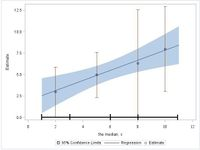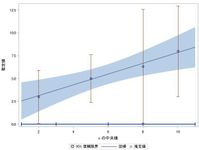## How Can I Insert Custom Quantile Line in SGPLOT Graph?

Hi, All:

I want to know How to Insert Custom Quantile Line to SGPLOT Graph.

Example code is following.

``````data test;
input x y @@;
datalines;
10 10 4 5 2 5 2 4 8 4 9 6 7 6 5 2
1 1 3 2 4 7 6 7 8 9 11 8 6 4
;
run;
proc univariate data=test noprint;
var x;
output out=pctl pctlpts=25 50 75 100 pctlpre=pctl;
run;
data test_1;
set test;
if _n_ eq 1 then set pctl;
if x le pctl25 then pctlgrp=1;
else if x le pctl50 then pctlgrp=2;
else if x le pctl75 then pctlgrp=3;
else if x le pctl100 then pctlgrp=4;
run;
proc sort data=test_1 out=test_2;
by pctlgrp;
run;
ods output basicintervals=mci(where=(parameter eq "Mean"));
proc univariate data=test_2 cibasic;
var y;
by pctlgrp;
run;
proc univariate data=test_2 noprint;
var x;
by pctlgrp;
output out=med median=med;
run;
data mci_1;
set mci(drop=varname parameter);
run;
data test_3;
merge test_2(in=a) med(in=b) mci_1(in=c);
by pctlgrp;
if a;
run;
proc sgplot data=test_3;
reg x=x y=y / clm nomarkers;
scatter x=med y=estimate / yerrorlower=lowercl yerrorupper=uppercl;
run;``````

On this graph, I want horizontal line (0th (x=1), 25th (x=3), 50th (x=6), 75th (x=8), 100th (x=11) percentile with marker line) on axis Y's "0.0", like following picture's black line.I tried to insert this line by making graph from specific data, but markers "PLUS" were out of this line's end.

Thank you for your kind cooperation.

1 ACCEPTED SOLUTION

Accepted Solutions

## Re: How Can I Insert Custom Quantile Line in SGPLOT Graph?

Have you tried the SG annotation feature?

First, you could include the 0th percentile in your PCTL dataset (see your first PROC UNIVARIATE step):

``output out=pctl pctlpts=0 25 50 75 100 pctlpre=pctl;``

Then use this dataset to supply coordinates to an SG annotation dataset:

``````%sganno; /* compile SG annotation macros */

data pctlline;
set pctl;
h=0.2; /* half the length of the vertical lines */
%sgline(x1=pctl0, y1=0, x2=pctl100, y2=0, drawspace="datavalue"); /* horizontal line */
%sgline(x1=pctl0, y1=-h, x2=pctl0, y2=h); /* first vertical line */
%sgline(x1=pctl25, x2=pctl25); /* subsequent vertical lines retain the same y-coordinates */
%sgline(x1=pctl50, x2=pctl50);
%sgline(x1=pctl75, x2=pctl75);
%sgline(x1=pctl100, x2=pctl100);
run;``````

(The SG annotation macros are available starting with the first maintenance release of SAS 9.4.)

``proc sgplot data=test_3 sganno=pctlline;``

I don't know why the vertical lines seem to be shifted a little bit to the right. If this is an issue, you may want to tweak their x-coordinates, e.g.:

``````data pctlline;
set pctlline;
x1=x1-0.02;
x2=x2-0.02;
run;``````

2 REPLIES 2

## Re: How Can I Insert Custom Quantile Line in SGPLOT Graph?

Have you tried the SG annotation feature?

First, you could include the 0th percentile in your PCTL dataset (see your first PROC UNIVARIATE step):

``output out=pctl pctlpts=0 25 50 75 100 pctlpre=pctl;``

Then use this dataset to supply coordinates to an SG annotation dataset:

``````%sganno; /* compile SG annotation macros */

data pctlline;
set pctl;
h=0.2; /* half the length of the vertical lines */
%sgline(x1=pctl0, y1=0, x2=pctl100, y2=0, drawspace="datavalue"); /* horizontal line */
%sgline(x1=pctl0, y1=-h, x2=pctl0, y2=h); /* first vertical line */
%sgline(x1=pctl25, x2=pctl25); /* subsequent vertical lines retain the same y-coordinates */
%sgline(x1=pctl50, x2=pctl50);
%sgline(x1=pctl75, x2=pctl75);
%sgline(x1=pctl100, x2=pctl100);
run;``````

(The SG annotation macros are available starting with the first maintenance release of SAS 9.4.)

``proc sgplot data=test_3 sganno=pctlline;``

I don't know why the vertical lines seem to be shifted a little bit to the right. If this is an issue, you may want to tweak their x-coordinates, e.g.:

``````data pctlline;
set pctlline;
x1=x1-0.02;
x2=x2-0.02;
run;``````

## Re: How Can I Insert Custom Quantile Line in SGPLOT Graph?

Hi, FreelanceReinhard: